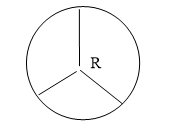Moment of intertia
Question

# A wheel comprises of a ring of radius R and M and three spokes of mass m each moment of inertia of the wheel about its axis is:Easy
Solution

## Moment of inertia of the ring is ${\mathrm{MR}}^{2}$  and that of each spoke is $\frac{{\mathrm{mR}}^{2}}{3}$ .Total moment of inertia  ${\mathrm{MR}}^{2}+\frac{{\mathrm{mR}}^{2}}{3}×3=\left(\mathrm{M}+\mathrm{m}\right){\mathrm{R}}^{2}$

Get Instant Solutions